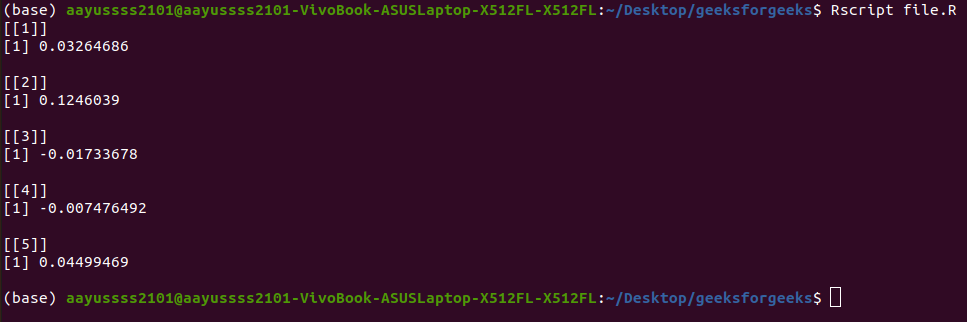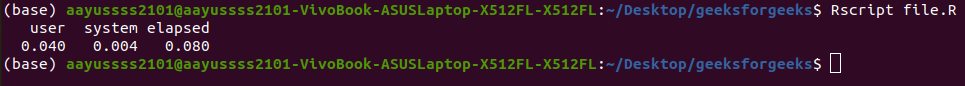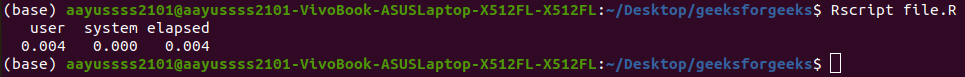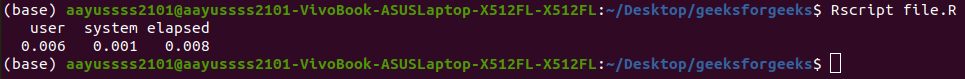Open in App
Not now

# Parallel processing using “parallel” in R

• Last Updated : 23 Jan, 2023

Parallel processing allows your application to do more tasks in less time. These assist in solving significant issues. In this article, we are going to look at how we can do parallel processing using the parallel library in R Programming Language.

## Using parallel library

The parallel is a base package that was included in R 2.14.0. It expands on the work done for CRAN packages multicore and snow and offers drop-in substitutes for the majority of those packages’ capabilities,

You can import the library using the following command:

`library(parallel)`

You can also check the number of cores in your system using the library. The following code does the same:

## R

 `library``(parallel)``detectCores``()`

Output:

`8`

## Methods of Parallelisation

The code can be parallelized primarily in one of two ways: either using sockets or by forking.

### Using sockets with parLapply()

This kind of parallel processing is slower and more difficult to use. The standard procedure we’ll use is as follows:

• Start an n-node cluster.
• Run any necessary pre-processing code in each node.
• In place of *apply, use par*apply.
• Destroy the cluster.

Let us go through an example to understand this better. For demonstration purposes, I am going to find the squares and cubes of numbers 1 to 5.

The following code does the job:

## R

 `lapply``(1:5, ``function``(x) ``median``(``rnorm``(x*100)))`

Output:If we will measure the time it takes to run this, we will get:

## R

 `time_taken <- ``system.time``(``    ``lapply``(1:5, ``function``(x) ``c``(x^2,x^3)) ``)`` ` `time_taken`

Output:Now let’s do the same task in a parallel fashion. The following code makes use of the parallel library to perform the same operation:

## R

 `library``(parallel)``  ` `no_cores <- ``detectCores``()``  ` `# Creating a cluster with the number of cores``clust <- ``makeCluster``(no_cores)`` ` `# Using parLapply instead of lapply``parLapply``(clust,``          ``1:5, ``function``(x) ``median``(``rnorm``(x*100)))`

Output:If we now measure the time it takes to execute we will get:

## R

 `library``(parallel)`` ` `no_cores <- ``detectCores``()``  ` `clust <- ``makeCluster``(no_cores)`` ` `time_taken <- ``system.time``(``    ``parLapply``(clust,1:5, ``function``(x) ``median``(``rnorm``(x*100)))``)`` ` `time_taken`

Output:As you can notice the second method takes a reduced amount of time than the first method.

### Forking with mclapply

In this approach, I am going to make use of the mclapply() method. We will go through the same example we have used in the above section, however, here we will be using mclapply() method instead of the lapply() method.

## R

 `library``(parallel)`` ` `mclapply``(1:5, ``function``(x) ``median``(``rnorm``(x*100)))`

Output:If we will now measure the execution time, we get:

## R

 `library``(parallel)`` ` `time_taken <- ``system.time``(``    ``mclapply``(1:5, ``function``(x) ``median``(``rnorm``(x*100))) ``)`` ` `time_taken`

Output:My Personal Notes arrow_drop_up